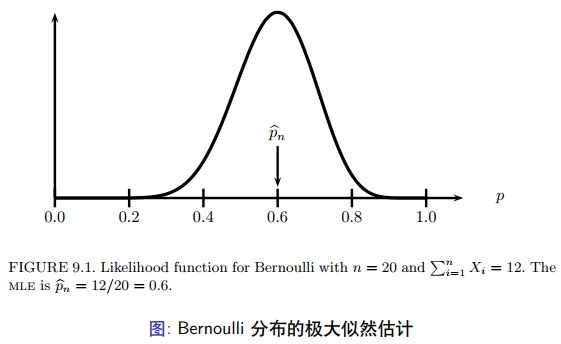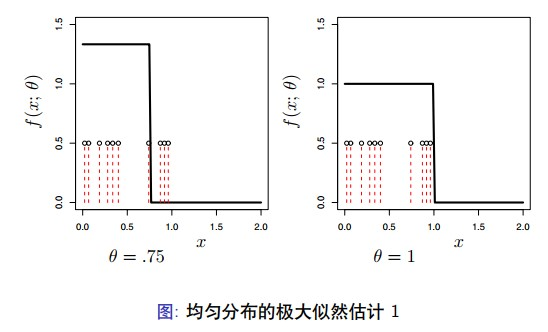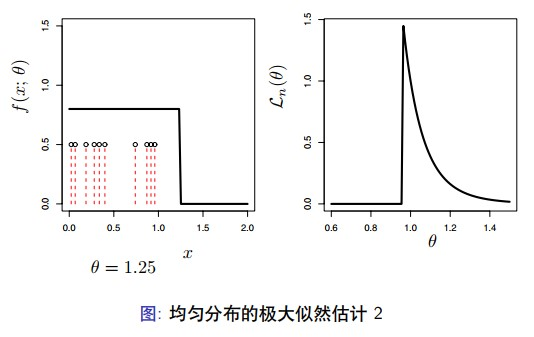# «统计学完全教程»笔记：第9章 参数推断

1. 有先验知识可以知道数据近似服从某种参数化模型。如，交通事故发 生的次数近似服从泊松分布。

2. 参数化模型的推断为理解非参数方法提供了背景知识。 这样, 我们还是要学习参数化模型。

## 矩估计

• 第一种用于参数估计的方法称为矩方法。我们将会看到，这些估计 器的并不是最优的, 但是他们却容易计算。这些方法得到的结果对 于需要迭代数值计算的方法是很好的初始值。

• 假定我们有参数 $\theta=\left(\theta_{1}, \ldots, \theta_{k}\right)$ 包括 $k$ 个成分。对于 $1 \leq j \leq k$, 定义 $j$ 阶矩 (Moment) 为 $$\alpha_{j} \equiv \alpha_{j}(\theta)=\mathbb{E}{\theta}\left(X^{j}\right)=\int x^{j} d F{\theta}(x)$$

• 同时, 定义第 $j$ 阶样本矩 (Sample Moment) 为 $$\widehat{\alpha}{j}=\dfrac{1}{n} \sum{i=1}^{n} X_{i}^{j}$$

1. 估计器 $\hat{\theta}_{n}$ 存在的概率趋近于 1 。

2. 这个估计器是一致估计, 即 $\widehat{\theta}_{n} \stackrel{\mathrm{P}}{\longrightarrow} \theta$ 。

3. 这个估计是渐进正态的，即 $$\sqrt{n}\left(\widehat{\theta}{n}-\theta\right) \rightsquigarrow N(0, \Sigma)$$ 其中 $$\Sigma=g \mathbb{E}{\theta}\left(Y Y^{T}\right) g^{T}$$ 并且， $$Y=\left(X, X^{2}, \ldots, X^{k}\right)^{T}, g=\left(g_{1}, \ldots, g_{k}\right), g_{j}=\partial \alpha_{j}^{-1}(\theta) / \partial \theta$$ 定理中的第三个陈述可以用于寻找标准误差和置信区间。但是，可以采 用更为简单的 Bootstrap 方式。

## 极大似然估计## 极大似然估计的性质

• 一致性

• 等变性

• 渐近正态性

• 最优性

1. 极大似然估计是一致估计 (consistent)， $\widehat{\theta}{n} \longrightarrow{P}{\longrightarrow} \theta{\star}$, 其中 $\theta_{*}$ 表示参数 的真实值。

2. 极大似然估计是同变估计 (equivariant), 如果 $\widehat{\theta}{n}$ 是 $\theta$ 极大似然估计, 则 $g\left(\widehat{\theta}{n}\right)$ 就是 $g(\theta)$ 的极大似然估计。

3. 极大似然估计是渐进状态 (asympotically Normal) 的, $\left(\hat{\theta}-\theta_{\star}\right) / \widehat{\mathrm{se}} \rightsquigarrow N(0,1)$, 同样, 估计的标准差 $\widehat{S e}$ 可以分析求解得到。

4. 极大似然估计是渐进最优的、有效的。这意味着，在所有表现正常的 估计器中, 极大似然估计的方差最小, 至少在大样本情形下成立。

5. 极大似然估计是渐进的 Bayes 估计器。 注意: 并不是 MLE 总是最好的, 在一般情形下, 是最好的。如果模型 满足某些正则条件 (regularity conditions)。这些是函数 $f(x ; \theta)$ 满足平滑 性 (smoothness) 的基本条件。

$9.13$ 定理 假定 $\theta_{*}$ 表示参数 $\theta$ 的真实值。定义 $$M_{n}(\theta)=\dfrac{1}{n} \sum_{i} \log \dfrac{f\left(X_{i} ; \theta\right)}{f\left(X_{i} ; \theta_{\star}\right)}$$ 并且， $M(\theta)=-D\left(\theta_{\star}, \theta\right)$ 。假设 $$\sup {\theta \in \Theta}\left|M{n}(\theta)-M(\theta)\right| \stackrel{\mathrm{P}}{\longrightarrow} 0$$ 对于任意 $\epsilon>0$, $$\sup {\theta:\left|\theta-\theta{\star}\right| \geq \epsilon} M(\theta)<M\left(\theta_{\star}\right)$$ 如果假定 $\hat{\theta}{n}$ 为极大似然估计, 那么 $\widehat{\theta}{n} \stackrel{P}{\longrightarrow} \theta_{\star}$ 。

$9.14$ 定理 假定 $\tau=g(\theta)$ 是关于 $\theta$ 的函数。假定 $\widehat{\theta}{n}$ 是参数 $\theta$ 的极大似然估计。那 么, $\widehat{\tau}{n}=g\left(\widehat{\theta}{n}\right)$ 就是 $\tau$ 的极大似然估计。 证明: 假定 $h=g^{-1}$ 表示 $g$ 的反函数。那么， $\widehat{\theta}{n}=h\left(\widehat{\tau}{n}\right)$ ，对于任意 $\tau$ ， 似然函数 $\mathcal{L}(\tau)=\prod{i} f\left(x_{i} ; h(\tau)\right)=\prod_{i} f\left(x_{i} ; \theta\right)=\mathcal{L}(\theta)$ ， 其中 $\theta=h(\tau)$ 。因 此，对于任意 $\tau ， \mathcal{L}{n}(\tau)=\mathcal{L}(\theta) \leq \mathcal{L}(\widehat{\theta})=\mathcal{L}{n}(\widehat{\tau})$ 。

$9.15$ 例题 假设 $X_{1}, \ldots, X_{n} \sim N(\theta, 1)$ ，对于参数 $\theta$ 的极大似然估计 $\widehat{\theta}{n}=\bar{X}{n}$ 。假定 $\tau=e^{\theta}$ ，那么，参数 $\tau$ 的极大似然估计 $\widehat{\tau}=e^{\widehat{\theta}}=e^{\bar{x}}$ 。

$9.16$ 定义 评分函数定义如下， $$s(X ; \theta)=\dfrac{\partial \log f(X ; \theta)}{\partial \theta}$$ Fisher 信息量定义为 \begin{aligned} I_{n}(\theta) &=\mathbb{V}{\theta}\left(\sum{i=1}^{n} s\left(X_{i} ; \theta\right)\right) \ &=\sum_{i=1}^{n} \mathbb{V}{\theta}\left(s\left(X{i} ; \theta\right)\right) \end{aligned} 对于 $n=1$ 时，我们更倾向于记为 $I(\theta)$, 而不是 $I_{1}(\theta)$ 。可以证明， $\mathbb{E}{\theta}(s(X ; \theta))=0$ 。这样，我们可以得到， $\mathbb{V}{\theta}(s(X ; \theta))=\mathbb{E}{\theta}\left(s^{2}(X ; \theta)\right)$ 。事 实上, 我们可以进一步简化 $I{n}(\theta)$ 。

$9.17$ 定理 $I_{n}(\theta)=n I(\theta)$, 同时 \begin{aligned} I(\theta) &=-\mathbb{E}_{\theta}\left(\dfrac{\partial^{2} \log f(X ; \theta)}{\partial \theta^{2}}\right) \ &=-\int\left(\dfrac{\partial^{2} \log f(x ; \theta)}{\partial \theta^{2}}\right) f(x ; \theta) d x \end{aligned}

$9.18$ 定理 (极大似然估计的渐进正态性) 假设 se $=\sqrt{\mathbb{V}\left(\widehat{\theta}_{n}\right)}$ ，在适当的正则条件下，有下列等式成立：

1. se $\approx \sqrt{1 / I_{n}(\theta)}$, 且 $$\dfrac{\left(\widehat{\theta}_{n}-\theta\right)}{\text { se }} \leadsto N(0,1)$$

2. 令 $\widehat{\mathrm{se}}=\sqrt{1 / I_{n}\left(\widehat{\theta}{n}\right)}$, 则 $$\dfrac{\left(\widehat{\theta}{n}-\theta\right)}{\widehat{\mathrm{se}}} \leadsto N(0,1)$$

$9.19$ 定理 令 $$C_{n}=\left(\widehat{\theta}{n}-z{\alpha / 2} \widehat{\mathrm{se}}, \widehat{\theta}{n}+z{\alpha / 2} \widehat{\mathrm{se}}\right)$$ 那么， $\mathbb{P}{\theta}\left(\theta \in C{n}\right) \rightarrow 1-\alpha$ ，当 $n \rightarrow \infty$ 。 证明: 假定 $Z$ 表示服从标准正态分布的随机变量。那么， \begin{aligned} \mathbb{P}{\theta}\left(\theta \in C{n}\right) &=\mathbb{P}{\theta}\left(\widehat{\theta}{n}-z_{\alpha / 2} \widehat{\mathrm{e}} \leq \theta \leq \widehat{\theta}{n}+z{\alpha / 2} \widehat{\mathrm{se}}\right) \ &=\mathbb{P}{\theta}\left(-z{\alpha / 2} \leq \dfrac{\widehat{\theta}{n}-\theta}{\widehat{\mathrm{se}}} \leq z{\alpha / 2}\right) \ & \rightarrow \mathbb{P}\left(-z_{\alpha / 2}<z<z_{\alpha / 2}\right)=1-\alpha \end{aligned} 对于 $\alpha=.05 ， z_{\alpha / 2}=1.96 \approx 2$, 因此. $$\widehat{\theta}_{n} \pm 2 \widehat{\mathrm{se}}$$ 是渐进的 $95 %$ 的置信区间。

$9.20$ 例题 假定 $X_{1}, \ldots, X_{n} \sim$ Bernoulli $(p)$ 。极大似然估计是 $\widehat{p}{n}=\sum{i} X_{i} / n$, 并且, $f(x ; p)=p^{x}(1-p)^{1-x}$, $\log f(x ; p)=x \log p+(1-x) \log (1-p)$, $$s(X ; p)=\dfrac{X}{p}-\dfrac{1-X}{1-p}$$ 并且， $$-s^{\prime}(X ; p)=\dfrac{X}{p^{2}}+\dfrac{1-X}{(1-p)^{2}}$$ 这样, $$I(p)=\mathbb{E}_{p}\left(-s^{\prime}(X ; p)\right)=\dfrac{p}{p^{2}}+\dfrac{(1-p)}{(1-p)^{2}}=\dfrac{1}{p(1-p)}$$

$9.21$ 例题 令 $X_{1}, \ldots, X_{n} \sim N\left(\theta, \sigma^{2}\right)$, 其中 $\sigma^{2}$ 已知。评分函数 $s(X ; \theta)=(X-\theta) / \sigma^{2}$ 并且 $s^{\prime}(X ; \theta)=-1 / \sigma^{2}$ ，因此 $I_{1}(\theta)=1 / \sigma^{2}$ 。极大似 然估计是 $\widehat{\theta}{n}=\bar{X}{n}$ 。根据 $9.18$ 定理, $\bar{X}_{n} \approx N\left(\theta, \sigma^{2} / n\right)$ 。这种情况下，正 态渐进就是完全精确的。

$9.22$ 例题 假定 $X_{1}, \ldots, X_{n} \sim$ Poisson $(\lambda)$ 。那么, $\widehat{\lambda}{n}=\bar{X}{n}$ 。计算可以得到 $I_{1}(\lambda)=1 / \lambda$, 因此, $$\widehat{\mathrm{se}}=\dfrac{1}{\sqrt{n l}\left(\widehat{\lambda}{n}\right)}=\sqrt{\dfrac{\widehat{\lambda}{n}}{n}}$$ 因此, $\lambda$ 的 $1-\alpha$ 渐进置信区间为 $\widehat{\lambda}{n} \pm z{\alpha / 2} \sqrt{\hat{\lambda}_{n} / n}$ 。

$9.23$ 定理 如果 $\widehat{\theta}{n}$ 是极大似然估计， $\tilde{\theta}{n}$ 是其他任意估计，则 $$\operatorname{ARE}\left(\tilde{\theta}{n}, \hat{\theta}{n}\right) \leq 1$$ 因此，极大似然估计具有最小渐进方差，称极大似然估计是有效的、渐 进最优的。 这个结论是基于模型正确的假设。如果模型假设本身有误，极大似然估 计就不再是最优的。

## Delta 方法

$9.24$ 定理 Delta 方法 如果 $\tau=g(\theta)$ ， 其中， $g$ 是可微的，且 $g^{\prime}(\theta) \neq 0$ ， 则 $$\overbrace{\dfrac{\left(\widehat{\tau}{n}-\tau\right)}{\operatorname{Se}(\widehat{\tau})}} \rightsquigarrow N(0,1)$$ 其中， $\widehat{\tau}{n}=g\left(\widehat{\theta}{n}\right)$, 并且, $$\widehat{\operatorname{se}}\left(\widehat{\tau}{n}\right)=\left|g^{\prime}(\widehat{\theta})\right| \widehat{\operatorname{se}\left(\widehat{\theta}{n}\right)}$$ 因此, 如果 $$C{n}=\left(\widehat{\tau}{n}-z{\alpha / 2} \widehat{\operatorname{se}\left(\widehat{\tau}{n}\right)}, \widehat{\tau}{n}+z_{\alpha / 2} \widehat{\operatorname{se}\left(\widehat{\tau}{n}\right)}\right)$$ 那么， $\mathbb{P}{\theta}\left(\tau \in C_{n}\right) \rightarrow 1-\alpha$ ，当 $n \rightarrow \infty$ 。

## 多参数模型

(多参数的 Delta 方法) 假定 $\nabla g$ 在 $\widehat{\theta}$ 处的值为 0 。如果 $\widehat{\tau}=g(\widehat{\theta})$, 则 $$\dfrac{(\widehat{\tau}-\tau)}{\widehat{\operatorname{se}}(\widehat{\tau})} \leadsto N(0,1)$$ 其中， $$\widehat{\operatorname{se}}(\widehat{\tau})=\sqrt{(\widehat{\nabla} g)^{T} \widehat{J}{n}(\widehat{\nabla} g)}$$ 其中 $\widehat{J}{n}=J_{n}\left(\widehat{\theta}_{n}\right)$, 并且 $\widehat{\nabla} g$ 是 $\nabla g$ 在 $\theta=\widehat{\theta}$ 的估计。